Maybe your company is like ours, and you use Excel to help keep track of many things. Pretty much every department and every employee has to use Excel at least a little bit.

Are you concerned that you might have too many Excel novices who don't know what they are doing? Knowing how to build simple Excel formulas is a necessary skill for every user to have. At some point everyone will need to at least verify that the totals displayed on a spreadsheet are correct.

(By the way, we recommend basic online Excel training for all new employees. Here are a few tips to get you pointed in that direction.)

Not knowing how to work with formulas can be a big disadvantage for individual employees and ultimately for your organization. Below are a couple of examples of simple formulas in Microsoft Excel.## How to build simple Excel formulas

The most popular formula used in Microsoft Excel is addition. Addition is used to add the values from two or more cells to get an answer. In the example below, I would like a total in cell A3 that adds up both the numbers found in cells A1 and A2.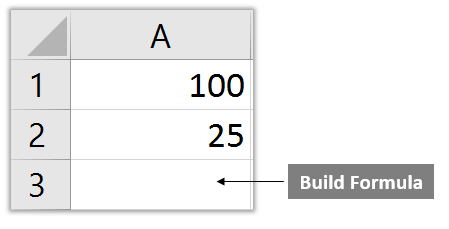### Steps for creating a simple Excel formula

1. Click in the cell where you would like to build the formula.
2. Type in the equals sign. All formulas must begin with an equals sign.
3. Click on the first cell you want to include in your formula. Notice the 'marching ants' around the cell.
4. Next, you will need to include an operator.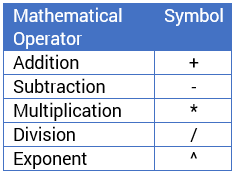5. After adding the operator, click on the second cell you would like to include in the formula. Later we will show you how to add a larger range of cells all at once.
6. Repeat steps 4 and 5 above to include data in additional cells.
7. Confirm the formula by hitting the enter key on your keyboard. Do not use the 'click away' method to enter your formula.

### Do use cell references

Always use the cell reference while building a formula (A1, A2 etc.). This will ensure that your formula totals will automatically update if any cell values are changed.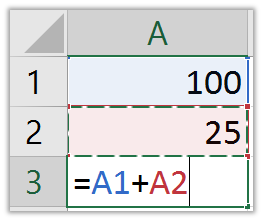### Don't use cell values

Do not enter the cell value while building a formula (100, 25 etc.). The formula will not automatically update if any cell values are changed.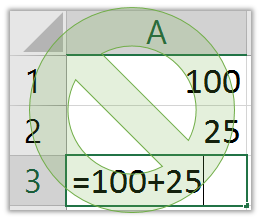## To view a formula

Even if you don't have to create a formula from scratch, knowing how to view a formula can help explain what type of formula was used, what cells are being referenced, and can also be used to audit a formula making sure that the correct range of cells is referenced. Formulas can be viewed a couple of different ways.

### The Formula Bar

Single click on a formula and look up in the formula bar to view the formula. Notice the = sign. All formulas begin with the equals sign.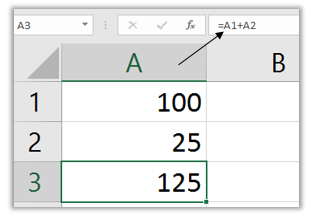### Double click on a formula

Double clicking on a formula displays a visual representation of the formula. Press the Esc (escape) key found in the upper left side of your keyboard to exit out of edit mode.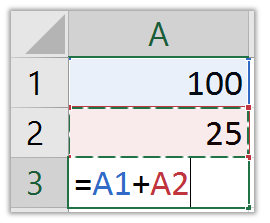## The SUM function

The SUM function is the most widely used function in Microsoft Excel. Functions are predefined formulas that are built-in to Microsoft Excel to make your life easier. (For example, I recently wrote about a few simple, handy date functions.) Fill in the required inputs and Microsoft Excel will do the rest.

In the example above we added two cell values using a formula that totaled them together. This time we are looking to add 6 cell values together. This is where using a function will save time over building a formula. Instead of typing the plus sign and clicking on each cell, will be able to click a button, confirm the correct range of cells has been selected, and then hit the enter key.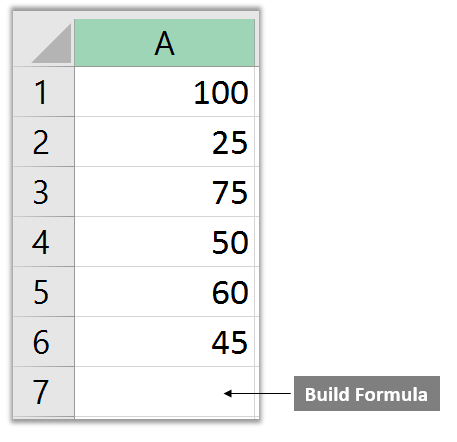### Steps for building the SUM function

• Click in the cell where you would like to build the formula.
• Click the AutoSum button located on the Home tab and Editing group.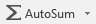• Hit the enter key to accept the formula. If the selected range is not correct, click and drag over a different block of cells, then hit the enter key.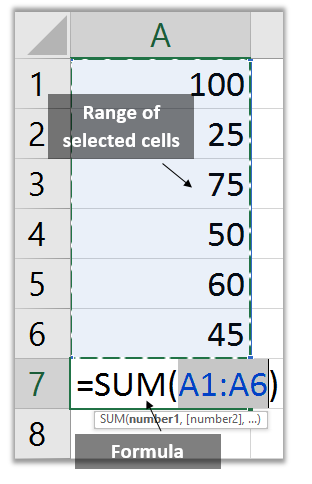### Breakdown of the SUM function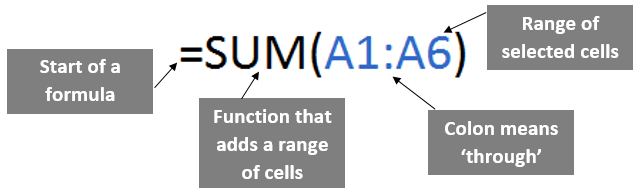Additional Excel functions can be found by clicking on the Fx button located next to the formula bar.

## Next steps

Need basic Excel training for your employees? KnowledgeWave offers online videos as well as live classes. It's a really affordable way to get training for everbody who touches Excel.Topics: Excel, Training